# Math in Focus Grade 1 Chapter 13 Answer Key Addition and Subtraction to 40

Practice the problems of Math in Focus Grade 1 Workbook Answer Key Chapter 13 Addition and Subtraction to 40 to score better marks in the exam.

## Math in Focus Grade 1 Chapter 13 Answer Key Addition and Subtraction to 40

Put on Your Thinking Cap!

Challenging Practice

Fill in the circles with numbers.

Question 1.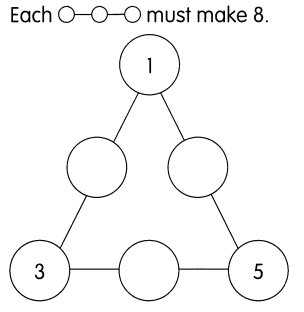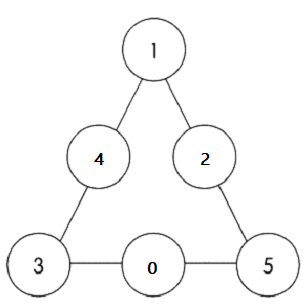Question 2.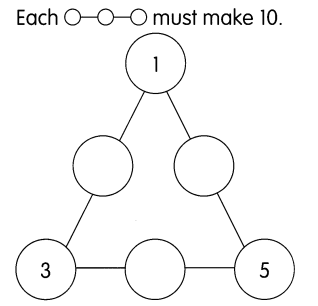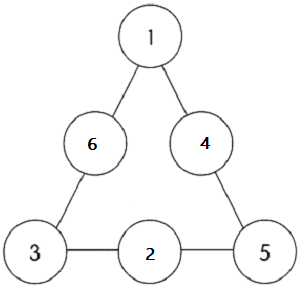Question 3.
Luis places a ball into the number machine below. What happens to the number on the ball? Fill in the missing numbers.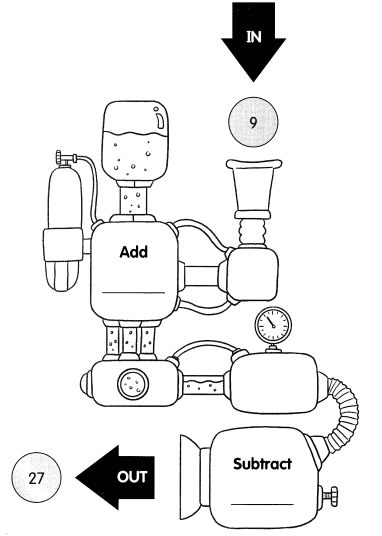Given,
Number 9 by adding 20 to 9 we get 29,
By subtracting 2 from 29 we get 27.

Question 4.
Luis places another ball into the number machine below. What happens to the number on the ball?
Fill in the missing numbers.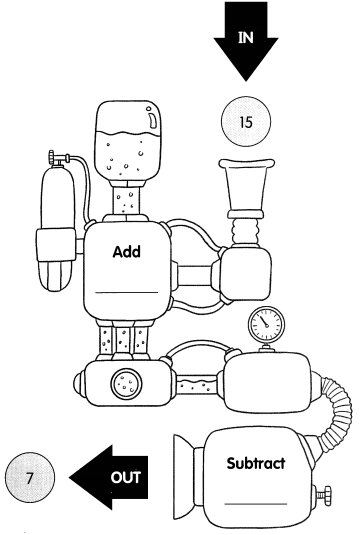Given,
Number 15 by adding 5 to 15 we get 20,
By subtracting 13 from 20 we get 7.

Put on Your Thinking Cap!

Problem Solving

Dawn, Jamal and Nate go to an amusement park.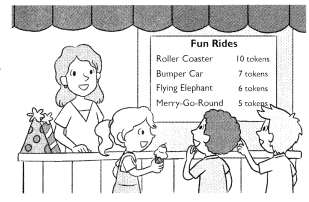They buy tokens. Then they go for rides. Which rides do they take?

Circle the correct rides.

Question 1.
Dawn uses 15 tokens to go on two different rides. Roller Coaster Bumper Car Flying Elephant Merry-Go-Round
Given,
Dawn uses 15 tokens to gon on two different rides,
Roller coaster ride is for 10 tokens,
Merry-Go-Round ride is 5 tokens,
By adding 10 with 5 we get 15,

Question 2.
Jamal uses 12 tokens to go on two different rides. Roller Coaster Bumper Car Flying Elephant Merry-Go-Round
Given,
Jamal uses 12 tokens to go on two different rides,
Bumper car ride is for 7 tokens,
Merry-Go-Round ride is for 5 tokens,
By adding 7 with 5 we get 12.

Question 3.
Nate uses 21 tokens to go on three different rides. Roller Coaster Bumper Car Flying Elephant Merry-Go-Round
Nate uses 21 tokens to go on three different rides,
Roller coaster ride is for 10 tokens,
Flying Elephant ride is for 6 tokens,
Merry-Go-Round ride is for 5 tokens,
By adding 10 with 6 we get 16 and then adding 16 with 5 we get 21.

Chapter Review/Test
Vocabulary
Choose the correct word.

Question 1.
You _________ from the greater number when you subtract.
Answer: You subtract smaller number from the greater number when you subtract.

Question 2.
You can _______ 13 ones into 1 ten 3 ones.
Answer: You can split 13 ones into 1 ten 3 ones.

Question 3.
You can add two numbers using the _______ method.
Answer: You can add two numbers using the sum method.

Question 4.
A _____________ can be used to add numbers.

Concepts and Skills

Question 5.
32 + 7 = ______

Question 6.
18 + 19 = ______

Question 7.
27 – 3 = ______

Question 8.
36 – 18 = ______

Question 9.
4 + 8 + 6 = ______

Question 10.
9 + 8 + 5 = ______

Problem Solving

Solve.

Question 11.
Nicole blows up 30 balloons for a class party. Michael blows up 4 fewer balloons than Nicole. How many balloons does Michael blow up?
Michael blows up ___ balloons.